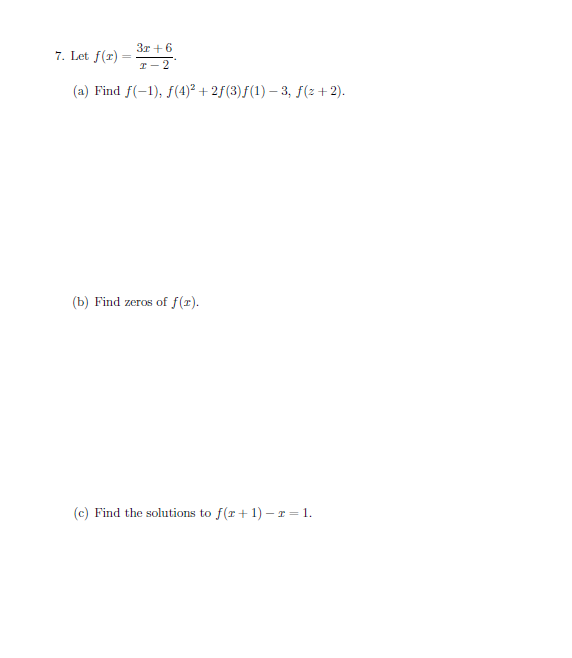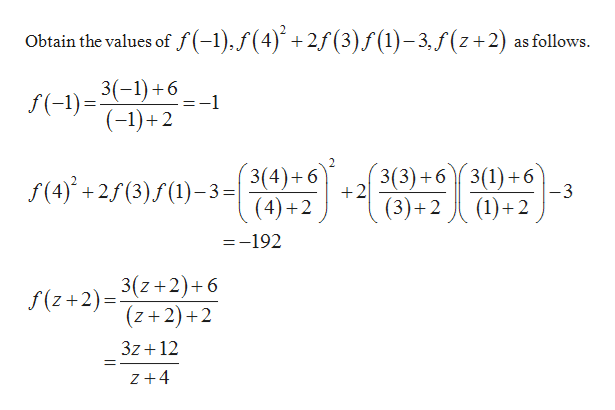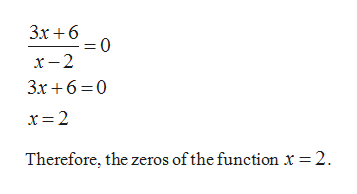# 3r67. Let f(rT-2(a) Find f(-1), f(4)2 + 25(3) f(1)-3, f(z +2)(b) Find zeros of f (x)(c) Find the solutions to f(r1-r = 1

Question

question is attached to image belowhelp_outlineImage Transcriptionclose3r6 7. Let f(r T-2 (a) Find f(-1), f(4)2 + 25(3) f(1)-3, f(z +2) (b) Find zeros of f (x) (c) Find the solutions to f(r 1-r = 1 fullscreen
check_circleExpert Solution
Step 1

The function is f(x) = (3x + 6) / (x – 2).

(a)help_outlineImage TranscriptioncloseObtain the values of f(-1),/(4)+2f(3) f (1)-3, f(z+2) as follows. 3(-1) 6 =-1 f(-1) (-1)+2 3(4)+6 42 3(3)6 3(1)6 +2 f(4)2(3)(1)3= -3 (1)2 (3)+2 =-192 3(z+2)+6 (z+2)+2 f(z+2)= 3z 12 Z+4 fullscreen
Step 2

(b)

Obtain the zeros of f(x) as...help_outlineImage TranscriptioncloseЗх +6 0 = х — 2 3x6 0 x 2 Therefore, the zeros of the function x = 2 fullscreen

### Want to see the full answer?

See Solution

#### Want to see this answer and more?

Solutions are written by subject experts who are available 24/7. Questions are typically answered within 1 hour*

See Solution
*Response times may vary by subject and question
Tagged in

### Other